# Cartan-Brauer-Hua theorem

Jump to: navigation, search
This page describes additional conditions under which a subgroup property implication can be reversed, viz a weaker subgroup property, namely Normal subgroup (?), can be made to imply a stronger subgroup property, namely central subgroup
View other subgroup property implication-reversing conditions

## Statement

Let$K < L$ be division rings (i.e., skew fields), with$K$ properly contained in$L$. Suppose$K^*$ is a normal subgroup of$L^*$. Then$K^*$ is a central subgroup of$L^*$, viz$K^*$ is contained in the center of$L^*$. In particular, this implies that$K^*$ is abelian and hence$K$ is a field.

## Proof

This proof uses a tabular format for presentation. Provide feedback on tabular proof formats in a survey (opens in new window/tab) | Learn more about tabular proof formats|View all pages on facts with proofs in tabular format

### Any element inside commutes with any element outside

For nonzero elements$x,y \in L^*$, we denote by$[x,y]$ the multiplicative commutator$xyx^{-1}y^{-1}$ and by$\! c_x(y)$ the element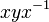$xyx^{-1}$.

We denote by$c_x$ the map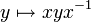$y \mapsto xyx^{-1}$. Here,$x \in L^*$ but$y$ is allowed to be zero.

Given:$g \in K^*$ and$a \in L \setminus K$.

To prove:$[g,a] = 1$.

Proof: The key idea is to play off the additive and the multiplicative structure against each other, and use the fact that the map$y \mapsto xyx^{-1}$ is an automorphism of both the additive and the multiplicative structure.

Step no. Assertion/construction Given data/assumptions used Previous steps used Explanation Commentary
1$g,a,a+1$ are all in$L^*$, so the notations$c_g$,$[g,a]$, and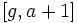$[g,a+1]$ make sense.$g \in K^*,a \in L \setminus K$. [SHOW MORE] The choice of$a$ and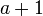$a + 1$ allows us to play on addition.
2$\! [g,a] \in K^*$ and$\! [g,a+1] \in K^*$.$K^*$ is normal in$L^*$ Step (1) [SHOW MORE]
3$\! c_g(a) = [g,a]a$ and$\! c_g(a + 1) = [g,a+1](a + 1)$ Step (1) [SHOW MORE] The multiplicative commutator is not convenient because it is not additive/linear in either variable. So, we rewrite it in terms of$c_g$, which preserves the additive structure.
4$c_g(a + 1) = c_g(a) + 1$ Step (1) [SHOW MORE] More manipulation.
5$\! [g,a+1](a+1) = [g,a]a + 1$, or equivalently,$\! ([g,a+1] - [g,a])a = 1 - [g,a+1]$ Steps (3) and (4) [SHOW MORE] More manipulation.
6 The assumption$\! [g,a+1] \ne [g,a]$ would lead to a contradiction, hence we must have$\! [g,a+1] = [g,a]$.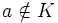$a \notin K$. Also, every nonzero element is invertible because$L$ is a skew field. Steps (2), (5) [SHOW MORE] More manipulation.
7 Plugging$[g,a+1] = [g,a]$ in the result of step (5) gives$[g,a] = 1$. Step (5) [SHOW MORE]

### The finishing touch

Now, if$K$ is a proper subset of$L$, we will show that$K^*$ is contained inside the center. We already know that every element of$K^*$ commutes with every element of$L \setminus K$. So it suffices to show that any two elements of$K^*$ commute.

Let$g,h \in K^*$. Then take any$a \in L \setminus K$. Then,$a + h \in L \setminus K$. Thus,$g$ commutes with both$a+h$ and$a$. Hence$g$ must commute with the difference, which is$h$.